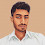# Puzzle : if 111 = 09 444 = 12 777 = 15 then 888 = ???Puzzle !!! :)
if
111 = 09
444 = 12
777 = 15
then
888 = ???

1.